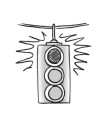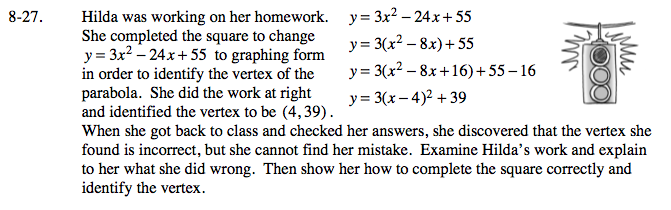### Home > A2C > Chapter 8 > Lesson 8.1.2 > Problem8-27

8-27.

Hilda was working on her homework. She completed the square to change y = 3x2 − 24x + 55 to graphing form in order to identify the vertex of the parabola. She did the work at right and identified the vertex to be (4, 39). When she got back to class and checked her answers, she discovered that the vertex she found is incorrect, but she cannot find her mistake. Examine Hilda's work and explain to her what she did wrong. Then show her how to complete the square correctly and identify the vertex. Homework Help ✎y = 3x2 − 24x + 55
y = 3(x2 − 8x) + 55
y = 3(x2 − 8x + 16) + 55 −16
y = 3(x − 4)2 + 39The error is in the step where she subtracted 16.

She should have subtracted 3 · 16 = 48 in order to account for the factor of 3.